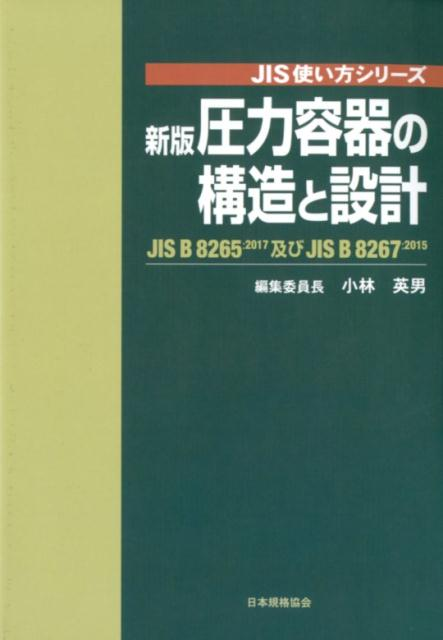PR

# Capacity of 10% head plate by hand calculation

In this article, we will analyze the shape of the head plate. Very often used in chemical plants.

This is because there are many facilities that operate at pressures other than atmospheric pressure, such as pressurized negative pressure.

When installing such equipment, it is necessary to calculate the nominal capacity firmly, including the application for fire protection.

In practice, it can be calculated by looking at the manufacturer’s HP or catalog. A formula is given. For example, look at the Hokkai site and you’re done.

In fact, the capacity formula can be analyzed within the scope of high school mathematics.

If you want to understand the principles in depth, why not try doing some calculations?

## Shape of 10% dish-shaped head plate

Let’s check the shape of the 10% dish-shaped end plate.

The model is below.

It has a mirror shape in which a large circle called R and a small circle called r are superimposed.

Here, the following relationship is presented for the mirror diameter D:

10% dish- shaped features

• R=D
• r=0.1D
• h=0.194D

Checking this relationship on drawings and calculating capacity is one of the practical tasks of mechanical engineers at chemical plants.

By the way thish = 0.194D _Do you know how it was derived?

### The relationship between the origin of R and r

First, we need to understand the relationship between R and r.

Mathematically, we have the following relationships:

1. R and r meet at contact point A
2. At the point of contact A, the curve of R and the curve of r have the same coordinates and slope
3. The origin of r is on a straight line connecting the origin of R and the point of contact A.

In particular, number 3, “The origin of r lies on a straight line connecting the origin of R and the point of contact A” should surprise you.

This is not listed in the catalog of the mirror plate.

If you know this relationship, h = 0.194D can be calculated easily, so why not post it?

It’s a little strange.

Let’s actually check the height of the panel.

“The origin of r is on the straight line connecting the origin of R and the point of contact A” is effective.

See the diagram below.

Consider the x,y coordinates with the origin O of R at (0,0).

The coordinates of the point of contact A between R and r are$$(D\cosΘ,D\sinΘ)$$is. This is the very definition of Θ.

Using this, the coordinates of r and the point of contact B on the barrel are

$$(D\cosΘ+0.1D(1-\cosΘ),D\sinΘ-0.1D\sinΘ)$$

It’s a little confusing, isn’t it?

If you know that the relationship between coordinate A and coordinate B is connected by a circle with radius r, you can easily derive it.

$$D(\cosΘ,\sinΘ)+0.1D(1-\cosΘ,\sinΘ)$$

It’s easier to see if you write

It’s a simple relationship.

Focusing on the relationship that “the coordinate of B is equal to the radius of the straight body”,

$$D\cosΘ+0.1D(1-\cosΘ)=0.5D$$

Since the relationship is obtained from here,

$$\cosΘ=\frac{4}{9},\sinΘ=\frac{\sqrt{65}}{9}$$

relationship is obtained.

difference between the y-intercept of the R curve and the y-coordinate of point B

$$h=D-D\sinΘ+0.1D\sinΘ$$

Tos i n ΘSubstituting the value of

$$h=0.194D$$

relationship is obtained.

The head volume calculation is only a calculation concept.

Volume calculation is integral

$$\int_{y1}^{y2}\pi x^2dy+\int_{y2}^{y3}\pi x^2dy$$

derived from

The reason for splitting the terms into two is, of course, that we have an R-curve and an r-curve.

The R-curve and r-curve have the following relationships, respectively.

$$x^2+y^2=R^2$$

$$(x-0.9D\cosΘ)^2+(y-0.9D\sinΘ)^2=r^2$$

From here, if you transform the formula for x and solve it, it will be derived.

As expected, this calculation is limited to my student days.

## Fundamentals of Pressure Vessel Design

Designing for mirrors is almost exclusively limited to designing pressure vessels.

This book is useful.created by Rinker
¥5,060(2023/09/29 17:30:33時点 楽天市場調べ-詳細)

## lastly

I introduced the shape analysis of the 10% dish-shaped end plate.

We introduce how to derive the mirror height h=0.194D from R=D, r=0.1D.

It also introduces the formula to calculate the volume of the mirror.